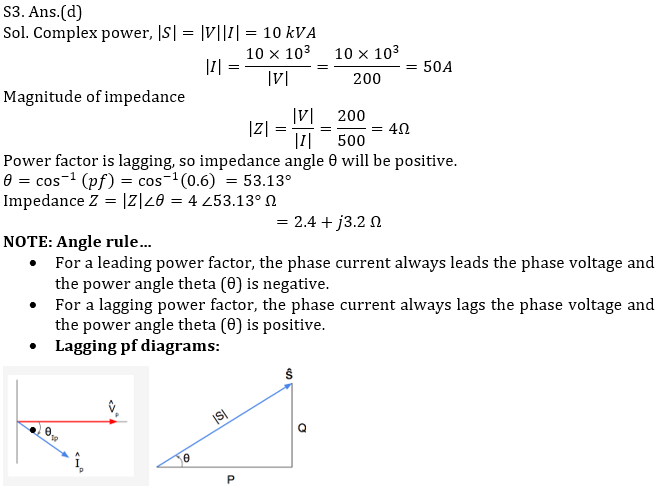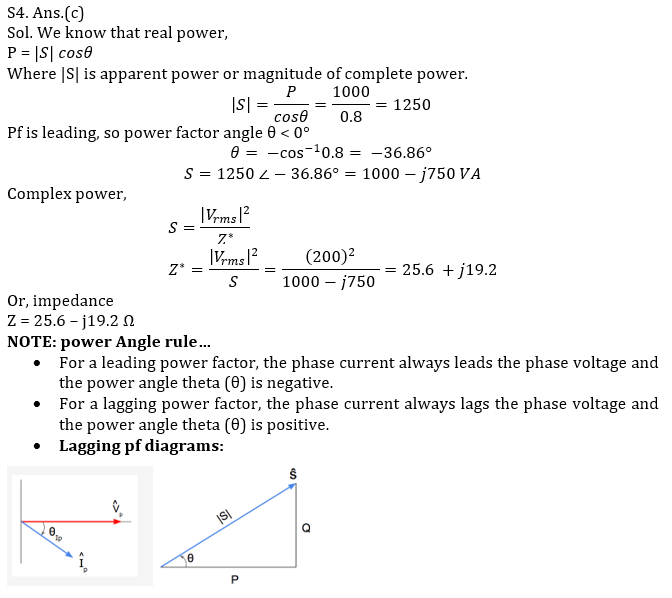Engineering Jobs   »   ELECTRICAL GATE QUIZ

# GATE’22 EE: Daily Practices Quiz 23-July-2021

Q1-Q2 Carry 1 mark each
Negative marking: 0.33 mark
Q3-Q5 carry 2 marks each
Negative marking: 0.66 mark

Total Questions: 05
Total marks: 8
Time: 12 min.

Q1. A single phase, full-wave, fully controlled bridge converter for supplying high inductive load is fed by a voltage V_m sin⁡ωt. The average and rms value of output voltages are
(a) (2V_m)/π cos⁡α and V_m/√2
(b) V_m/π cos⁡α and V_m/√2
(c) (2V_m)/π cos⁡α and V_m
(d) V_m/π cos⁡α and √2 V_m

Q2. Which testing method is performed to determine No Load losses in DC motor?
(a) Running down test
(b) Field test
(c) Swinburne’s test
(d) Brake test

Q3. An electric device is rated 10 kVA, 200 V at a 0.6 power factor lag. What is the impedance of the device?
(a) 4+j4Ω
(b) 1.2+j1.6Ω
(c) 2.4-j3.2Ω
(d) 2.4+j3.2Ω

Q4. In an ac circuit the following quantities are known to us
Real power, P = 1000W
Power factor, pf = 0.8 (leading)
Rms voltage, V_rms= 200 V
What is the total impedance of the circuit?
(a) 32 – j24Ω
(b) 25.6 + j19.2 Ω
(c) 25.6 – j19.2 Ω
(d) 32 + j24Ω

Q5. Two capacitance, C1 = 150 ± 2.4µF and C2 = 120 ± 1.5 µF are connected in parallel. What is the limiting error of the resultant capacitance C (in µF)?
(a) 0.9 µF
(b) 2.4 µF
(c) 1.5 µF
(d) 3.9 µF

SOLUTIONS

S1. Ans.(a)
Sol. A single phase, full-wave, fully controlled bridge converter for supplying high inductive load: V_avg=(2V_m)/π cos⁡α
And V_rms=V_m/√2

S2. Ans.(c)
Sol. Swinburne’s test is the most commonly used and simplest method of testing of shunt and compound wound DC machines which have constant flux.
In this method of testing no load losses are measured separately and eventually we can determine the efficiency.S5. Ans.(d)
Sol. Equivalent capacitance of the given parallel combination is C = C1 + C2. So, limiting error of C is (2.4 + 1.5) = 3.9 µF

Sharing is caring!